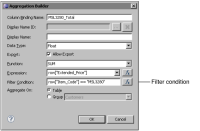Filtering aggregate data
When calculating aggregate data, you can specify a filter condition to determine which rows to factor in the calculation. For example, you can exclude rows with missing or null credit limit values when calculating an average credit limit, or include only deposit transactions when calculating the sum of transactions.
To specify a filter condition when aggregating data, in Aggregation Builder, specify a filter expression that evaluates to true or false. The following examples of aggregations include filter conditions:If summing the values in the Extended_Price field, adding the following filter expression returns the sum of extended prices for item MSL3280 only:
row["itemCode"] == "MSL3280"If averaging the values in the orderAmount field, adding the following filter expression returns the average order amount for closed orders only:
row["orderStatus"] == "Closed"
Figure 10‑12 shows an example of an aggregation in Aggregation Builder that includes a filter condition. The aggregation returns the sales total for item MSL3280.Figure 10‑12 Aggregation definition that includes a filter condition
Excluding null values from an aggregate calculation
When calculating the sum of a numeric field, it does not matter if some of the rows contain null values for the specified numeric field. The results are the same, regardless of whether the calculation is 100 + 75 + 200 or 100 + 75 + 0 (null) + 200. In both cases, the result is 375. Note that null is not the same as zero (0). Zero is an actual value, whereas null means there is no value.
Some aggregate calculations, however, return different results when null values are included or excluded from the calculation. The average value returned by the calculation without the null value in the previous example is 125, which is calculated as (100 + 75 + 200)/3. The average value of the calculation with the null value, however, is 93.75, which is calculated as (100 + 75 + 0 + 200)/4. Similarly, COUNT returns a different number of total rows, depending on whether you include or exclude rows with null values for a specified field.
By default, aggregate functions include all rows in their calculations. To exclude rows in which a specified field contains null values, specify a filter condition, as described in the following examples:If averaging the values in a transactionAmount field, adding the following filter condition performs the aggregate calculation on rows where the transaction amount is not null:
row["transactionAmount"] != nullIf counting the number of new customers in a report, adding the following filter condition counts only rows in which the creditLimit field has no value (indicating new customers):
row["creditLimit"] == null
Counting unique field values in a set of rows
A field can contain duplicate values. Sometimes, you want to get the count of distinct values. For example, a table displays a list of customers and their countries, as shown in Figure 10‑13. The table lists 12 customers from 4 different countries and a customer for which the country is not available.Figure 10‑13 A table that lists customers and their countries
If you insert a data element that uses COUNT in the header or footer row of the table, COUNT returns 12, the number of rows in the table. However, if you want to get the number of countries, use COUNTDISTINCT instead.
In the example report, COUNTDISTINCT returns 5, not 4 as you might expect, because like the other aggregate functions, COUNTDISTINCT counts rows with null values. The third row in the table contains a null value for country. To get the real count of countries that are listed in the table, add a filter condition to the aggregation, as follows:
row["country"] != null
This condition counts only rows in which the country value is unique and not null.